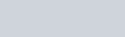Lorem ipsum dolor sit amet, consectetur adipiscing elit, sed do eiusmod tempor incididunt ut labore.

15 St Margarets, NY 10033
(+381) 11 123 4567
ouroffice@aware.com

### KMF## Why Should I Care?

Dynamic programming is the process of breaking down a larger problem into smaller problems. By using the answers to those smaller problems, we can find the overall solution more efficiently.

We’ll also learn about the term “memoization,” and how it relates to dynamic programming.

## How Does It Work?

The usual example of dynamic programming is the Fibonacci Sequence. It’s a good example, so we’ll use it here too. If you’re already familiar with the Fibonacci Sequence (and calculating it using recursion), then feel free to skip this next section.

If you’re not sure what that means, or you want a quick refresher, read on…

### The Fibonacci Sequence

This is a sequence of numbers, where each number is calculated by adding the previous two together:Imagine I ask you to programmatically calculate the 6th number in the sequence (which is `8`, as shown above).

How would you calculate it?

Here’s some JavaScript code for a function that does just that:

``````function f(n) {
// The first and second values are always 1
if (n == 1 || n == 2)
return 1;

// Calculate the previous two values in the sequence
// using this same function
return f(n - 1) + f(n - 2)
}
// Calculate the 6th value in the sequence

This will work fine, but it’s slow.

You can see that the function calls itself; it is recursive.

To calculate the sixth Fibonacci number, we first need to calculate the fourth and fifth Fibonacci numbers.

Each of those then have to calculate their previous numbers, and this repeats all the way down to the beginning of the sequence.

Here’s what that looks like if we draw it out as a graph.You can see that there’s a lot of duplication here. For example, the second value in the sequence is calculated five times!

For small numbers in the sequence, this isn’t too bad, but it quickly gets worse. For later numbers in the sequence, this would soon become impractical, even on a modern computer.

So how do we do better?

### Dynamic Programming and Memoization

One way we could improve this function is to store the results of our previous calculations as we go along. That way, we only need to calculate each number in the Fibonacci sequence once.

This is the essence of dynamic programming:

Dynamic programming is breaking the larger problem into smaller problems, and using those to get to the answer.

Because we’re achieving this by storing the previous results for later, we’re also using memoization:

Memoization is storing the result of a previous calculation so that we can use it later, to make the overall algorithm more efficient.

We could implement these concepts on the recursive approach above, but it’s easier to follow if we use a “bottom-up” approach.

Let’s look at the code first, and then we can discuss why this is called a bottom-up algorithm:

``````function f(n) {
// The first and second values are always 1
if (n == 1 || n == 2)
return 1

let result = 0

// Used to store the previous two results
let lastButOneValue = 1
let lastValue = 1

// Work from item 3 of the sequence (items 1 and 2 are a
// special case, see above), calculate each value in turn
for (let i = 3; i <= n; i++) {
// Calculate this number by adding together the
// previous two
result = lastValue + lastButOneValue
// Update the values of the
// previous two items in the sequence
lastButOneValue = lastValue        lastValue = result    }
return result
}
// Calculate the 6th value in the sequence

Here, we calculate the Fibonacci sequence in order ⁠— all the way from the beginning ⁠— until we get to the number in the sequence that we need.

As we go along, we store the results of the previous value, and the one before that.

Getting the next value is then trivial. Just add those two together.

Here’s the graph from the original (inefficient) algorithm again, but we’ve highlighted only the calculations we’re making in this new version:You can see that this time, rather than starting from the answer and working backward, we work forward until we reach the value we need.

When we visualize it, we’re working from the bottom of the graph upwards ⁠— hence a “bottom-up” approach.

This algorithm is much more efficient. There’s no duplication at all!

We learned some new terms here, so let’s recap.

## Recap

Our latest algorithm uses dynamic programming, because we’re breaking the bigger problem into smaller problems, and using their results to get to the overall answer.

It also uses memoization, because we’re storing the results of the previous step to avoid re-calculating them again later.

It was a bottom-up approach, because when we visualize the problem as a graph, we’re working from the base of the graph upwards (rather than top-down, as in the less efficient algorithm).

## Want to Know More?